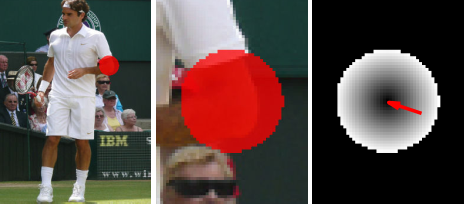# Towards Accurate Multi-person Pose Estimation in the Wild (CVPR 2017)Goal

George Papandreou, Tyler Zhu, Nori Kanazawa, Alexander Toshev, Jonathan Tompson, Chris Bregler, Kevin Murphy

## Overview

1. 基于回归的方法: 直接通过网络回归出图像中人体关键点的坐标, 这种方法在早期(2014年左右)有使用, 但由于回归任务很难训练, 现在(2020年)几乎看不到了
2. 基于分类的方法: 将人体关键点检测看作类似分割的任务, 通常在关键点处放置高斯核作为监督信号, 让网络输出热图(heatmap), 以表示关键点可能的位置及其概率, 再通过一些后处理的手段从热图得到关键点坐标. 这种方法中, 关键点位置实际上是一片区域, 而不仅仅是一个点, 网络容易学习. 不足之处就是由于最终热图的尺寸降采样很严重, 由量化误差导致的性能损失较多
3. 分类与回归相结合的方法: 据我所知, 本文应该是最早结合分类与回归的方法. 这种方法为每个位置预测当前位置到关键点的偏移量, 然后基于霍夫投票的思想, 把关键点附近的各个位置的偏移量的预测加和起来, 可以得到更准确的关键点位置. 但是由于本文没有开源代码, 而且霍夫投票的这个公式写的极难理解, 导致很多人只能通过文中图2去猜测实际的做法. 本篇博客旨在详细解析该论文的公式(1)

## Heatmap and Offset Prediction with CNN

### Network Output

$K$ 为关键点的数量, 网络的输出的channel数为 $3K$, 即每个关键点有3层输出. 其中1层是heatmap, 本文中是一个二值的mask. 其余两层是offset, 其值表示该位置在 $x$$y$ 方向与关键点的距离.

### Heatmap

Heatmap应该很好理解, 本文中使用的是二值的mask, 关键点周围圆形区域值为1, 其他位置值为0. Heatmap表示了关键点位置的粗略估计, 即关键点周围的一片圆形区域, 如上图中红色区域.

### Offset

Offset的ground-truth如上图的最右子图. Offset有两个channels, 这里画出的应该是两个channel的值的平方和的根, 也即欧式距离. 距离关键点越远, 值/距离越大, 但到了heatmap圆形区域外的部分忽略.

$f_{k}\left(x_{i}\right)=\sum_{j} \frac{1}{\pi R^{2}} G\left(x_{j}+F_{k}\left(x_{j}\right)-x_{i}\right) h_{k}\left(x_{j}\right)$

$f_{k}\left(x_{i}\right)$在文中被称为highly localized activation maps, 对应下图最右的fused activation maps. $x_{j}+F_{k}\left(x_{j}\right)$ 表示的是位置$x_{j}$对于关键点所在位置的预测, 把这些预测加和起来就实现了霍夫投票, 得到一个更为精细的关键点位置预测. 这里最令人费解的是$G(\cdot)$ -- the bilinear interpolation kernel, 文中完全没有对此的详细说明. 我猜测 $G(\cdot)$应该是一个输入2D向量, 输出一个标量的函数. 2D向量是$x_{j}+F_{k}$$x_{i}$的offset, offset越大, 输出值越接近0, 那么$f_{k}\left(x_{i}\right)$值越小, 表示$x_{i}$位置不太可能是关键点位置.